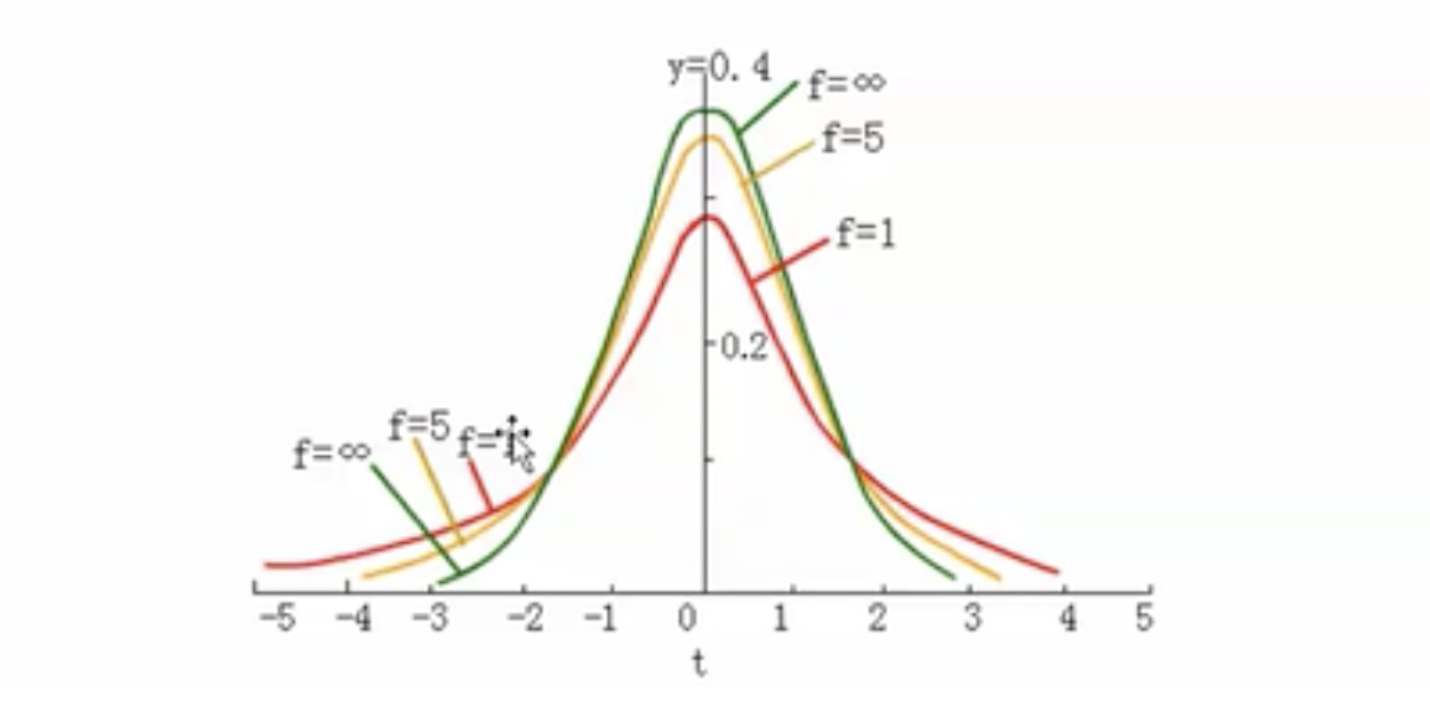$P(X=k) = 0.5, k=0,1$

$f(x) = {1 \over \sqrt{2\pi}\sigma}e^{-{(x-\mu)^2 \over 2\sigma^2}}, x \in (0,+\infty)$

$F(1.7 < x < 1.8) = \int_{1.7}^{1.8} f(x)dx$$P(|p - p\_| < 2 * {\sqrt{p * (1 - p) \over n}}) = 0.95$

$(p\_ - 2 * {\sqrt{p * (1 - p) \over n}},\enspace p\_ + 2 * {\sqrt{p * (1 - p) \over n}})$$0.95 = P(|{\bar{x} - \mu \over S / \sqrt{n}}| < t_{\alpha/2,n-1}) = P(\bar{x} - t_{\alpha/2,n-1} * {S \over \sqrt{n}} < \mu < \bar{x} + t_{\alpha/2,n-1} * {S \over \sqrt{n}}) \\ \text{其中：}\bar{x}\text{为样本均值， }\mu\text{为总体均值， }S\text{为样本标准差， }n\text{为试验次数}$

$\text{样本均值}\enspace\bar{x} = {1.7 + 1.6 + 1.8 + 1.72 + 1.75 + 1.74 \over 6} = 1.72 \\ n = 6,\enspace n-1 = 5 \\ \text{95\%的置信度时}\enspace \alpha = 0.05,\enspace t_{\alpha/2, 5} = 2.571 \\ s = \sqrt{(1.7 - 1.72)^2 + (1.6 - 1.72)^2 + (1.8 - 1.72)^2 + (1.72 - 1.72)^2 + (1.75 - 1.72)^2 + (1.74 - 1.72)^2 \over 5} = 0.0612 \\ \text{总体均值估计区间为：}\enspace(\bar{x} - t_{\alpha/2,n-1} * {S \over \sqrt{n}}, \bar{x} + t_{\alpha/2,n-1} * {S \over \sqrt{n}}) = (1.650, 1.790)$